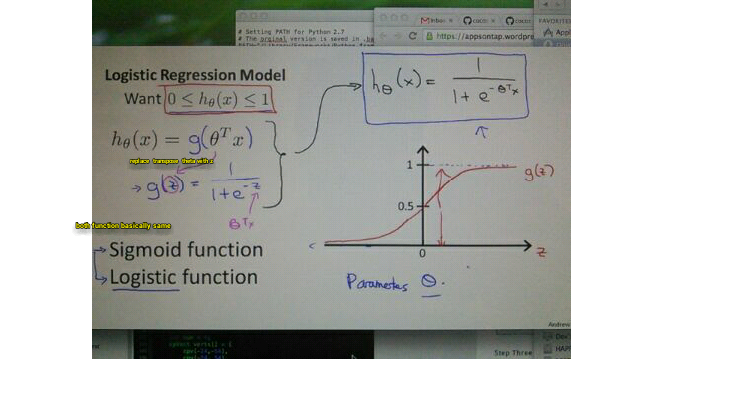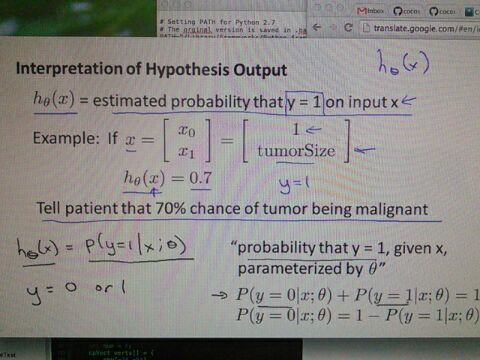the target is to build :the hypothesis must be between zero and one.

hypothesis for logistic regression modified as stated above.

Sigmoid and logistic function basically are the same thing.

we're going to replace theta transpose with z.

more z approach towards infinity ,  g(z)  approach 1
the same as  z  negative towards infinity,  g(z)  approach 0default y = 1
so if h0(x) = 0.7 then y = 1;
70% of being malignant
the default for x0 is as usual,  1
and x1 is a feature of tumorsize

the probability are whether y is 0 or 1 (both probability equal 1)

in summary,  the lesson here is about to introduce hypothesis function,  mathematical in logistic regression...